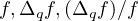Electron. J. Differential Equations, Vol. 2020 (2020), No. 14, pp. 1-14.

### Existence of rational solutions for q-difference Painleve equations Hong Yan Xu, Jin Tu

Abstract:
This article studies properties of meromorphic solutions for several types of q-difference Painleve equations. We obtain conditions for the existence, and the form of rational solutions for two classes of q-difference Painleve equations. Also for a solution f we obtain results about the fixed points, the exponents of convergence of poles of. Our results extend previous theorems given in the references.

Submitted January 13, 2019. Published February 5, 2020.
Math Subject Classifications: 39A13, 30D35.
Key Words: Rational solution; q-difference Painleve equation; fixed point.

Show me the PDF file (334 KB), TEX file for this article.Hong Yan Xu School of Mathematics and Computer Science Shangrao Normal University Shangrao Jiangxi 334001, China email: xhyhhh@126.com Jin Tu Department of Mathematics Jiangxi Normal University Nanchan, Jiangxi 330022, China email: tujin2008@sina.com